﻿ Heat Radiation of Plate (Laminar Flow) by Forced ConvectionExamples | Product | Murata Software Co., Ltd.# Example1Heat Radiation of Plate (Laminar Flow) by Forced Convection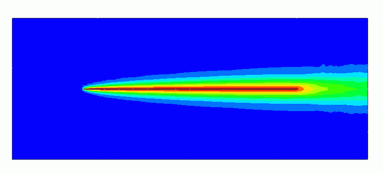General

• Heat dissipation of a flat plate by the forced convection is solved by the steady-state analysis.

• The temperature distribution and the heat flux vectors are solved.

• Unless specified in the list below, the default conditions will be applied.

• Obtain this session&apos;s project file. (Save the project file before open)

### Analysis Space

 Item Setting Analysis Space 2D   Thickness in depth direction: 300mm Model Unit mm

### Show Results

 Item Setting Solver Fluid Analysis [Bernoulli] Thermal analysis [Watt] Analysis Type Steady-State Analysis Laminar Flow/Turbulent Flow Select Laminar Flow Meshing Setup General Mesh size: 10[mm]

### Model

The material of Air (000_Air) is set to a rectangular sheet body. The boundary conditions of inlet and outlet are set on the left edge and the right edge respectively.

A heat source of thin flat plate is defined by the rectangle sheet body.

The slip wall outer boundary condition is applied to the top and bottom edges where the boundary condition is not set.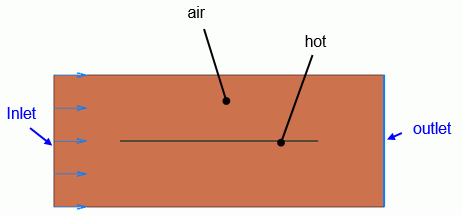### Body Attributes and Materials

 Body Number/Type Body Attribute Name Material Name 0/Solid air 000_Air(*) 1/Solid hot hot

* Available from the material DB

The material properties of the thin flat plate are set as follows.

 Material Name Tab Properties hot Solid/Fluid Solid Thermal conductivity 1x 10*5

The heat source of the thin flat plate is set on the heat source tab as follows.

 Body Attribute Name Tab Setting hot Heat source 10W

### Boundary Condition

 Boundary Condition Name/Topology Tab Boundary Condition Type Setting Inlet/Edge Thermal Fluid Inlet Forced Inflow Specify fluid velocity 0.1[m/s] Inflow Temperature : 0[deg] Outlet/Face Thermal Fluid Outlet Natural Outflow Outer Boundary Condition Thermal Fluid Slip wall –

It is said that the flow over the flat plate transitions to the turbulent flow at the Reynolds number of around 5×10^5

The Reynolds number calculated from this model form, material property, and fluid velocity is about 1986. It is small enough to analyze the laminar flow.

Viscosity μ=1.82e-5[Pa s]

Density ρ: 1.205[kg/m3]

Kinematic viscosity v=μ/ρ=1.82e-5/1.205=1.510e-5[m2/s]

Fluid velocity V=0.1[m/s]

Length of plate L=0.3[m]

Reynolds number Re = V*L/ν=0.1*0.3/1.510e-5 = 1986

### Results

The temperature distribution is shown below.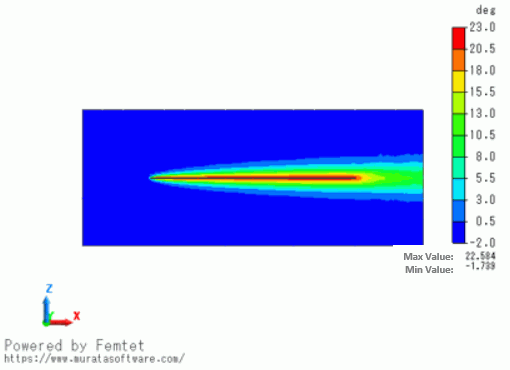The maximum temperature is 22.584 [deg].

The theoretical value of the heat transfer of the laminar flow is 24 [deg], which is close to the actual value.

<Theoretical Value Calculation>

Heat source Q=10 [W]

Thickness of plate W=0.3 [m]

Length of plate L=0.3 [m]

Average heat flux q=Q/(2*L*W)=55.6 [W/m2]

Viscosity μ=1.82e-5[Pa s]

Density ρ=1.205[kg/m3]

Kinematic viscosity v=μ/ρ=1.82e-5/1.205=1.510e-5[m2/s]

Thermal conductivity λ=0.0265[W/m/deg]

Specific heat Cp=1006[J/Kg/deg]

Temperature diffusivity α = λ/(ρ*Cp) = 2.19e-5 [m2/s]

Prandtl number Pr = ν/α = 0.691

Fluid velocity V = 0.1[m/s]

Laminar average heat transfer h = 0.664 λPr^1/3 ν^(-1/2) (V/L)^1/2 = 2.311 [W/m2/deg]

Temperature difference  ΔT = q/h = 24.04[deg]

Below is the plotting of the temperature distribution from coordinates (250,0,-100) to coordinates (250,0,100).

The boundary layer of about 30mm is created.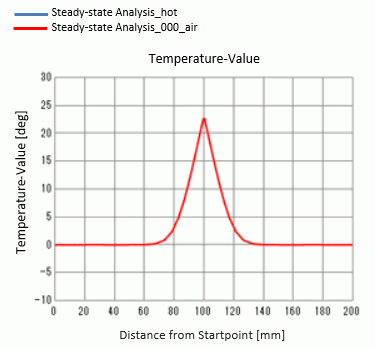The following is a vector diagram of the heat flux on the wall surface.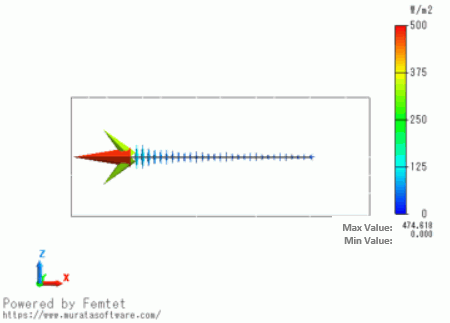The heat flux on the wall surface becomes larger toward the upwind.

For reference, the results of the turbulent analysis is shown below.

Almost the same results are acquired.

(In most cases of the laminar phenomena, the turbulent analysis can be applied.)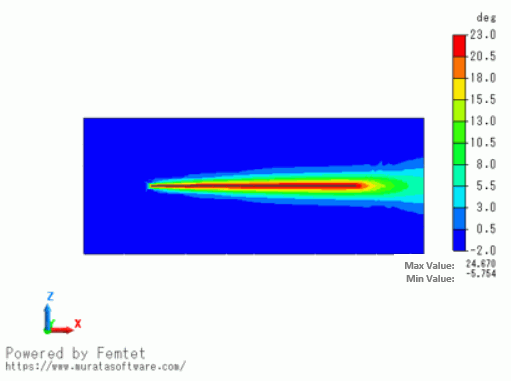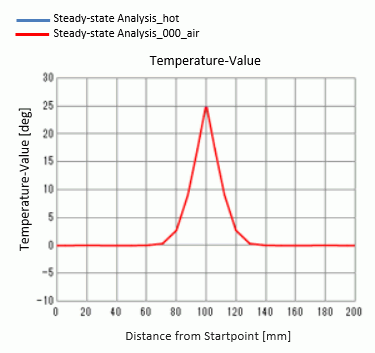﻿